Get instant live expert help with Excel or Google Sheets“My Excelchat expert helped me in less than 20 minutes, saving me what would have been 5 hours of work!”

#### Post your problem and you’ll get Expert help in seconds.

Your message must be at least 40 characters
Our professional Expert are available now. Your privacy is guaranteed.

# How To Calculate Percentages in Excel and Google Sheet

We can use Excel and Google Sheets to calculate percentage in many ways. In this tutorial, we will discover how to use the basic percentage formula, calculate percentages in Excel and explore different formulas for calculating percentage increase. We will also illustrate the excel percentage formula in multiple cells to calculate the percent increase or decrease.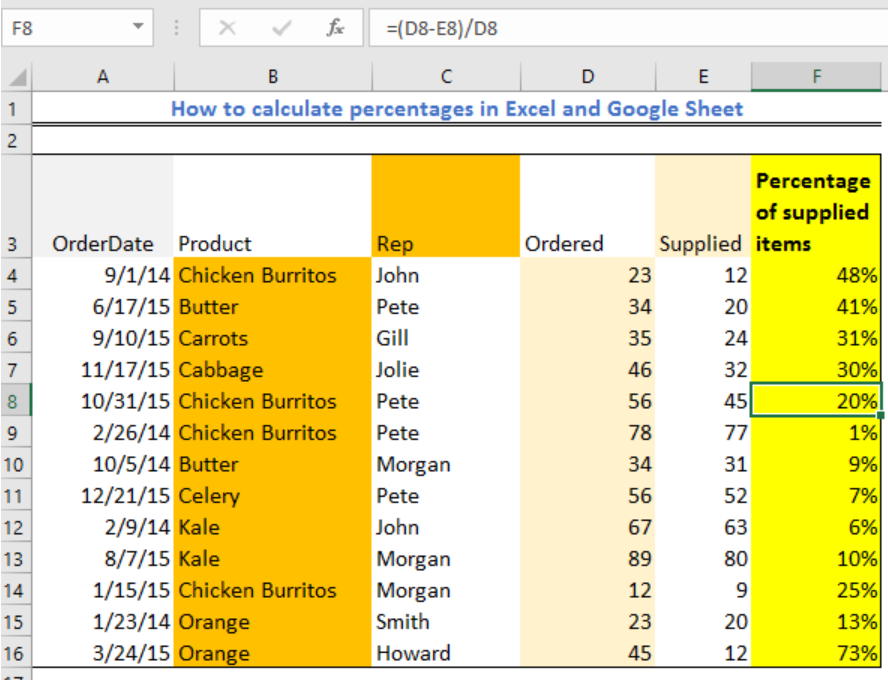Figure 1 – Percentage of a number in Excel

## General Formula

`New Value/Old Value = Percentage`

`(Old Value – New Value)/Old Value = Percent Change`

## How to Calculate Percentage Increase or (Change) Between Two Columns

We can quickly calculate the percentage change in our excel sheet across two columns using the steps below:

• We click on Cell F4 and enter the formula below

`=(D4-E4)/D4`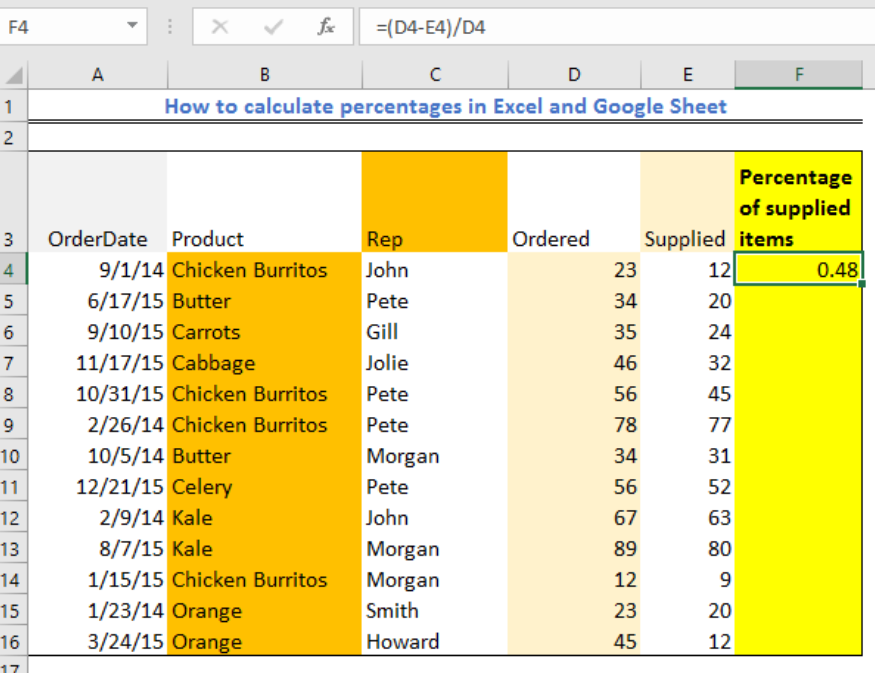Figure 2 – Drawing percentage in excel

• We will copy down the formula using the fill handle tool (the small box at the lower right corner of the cell) into the number of rows given.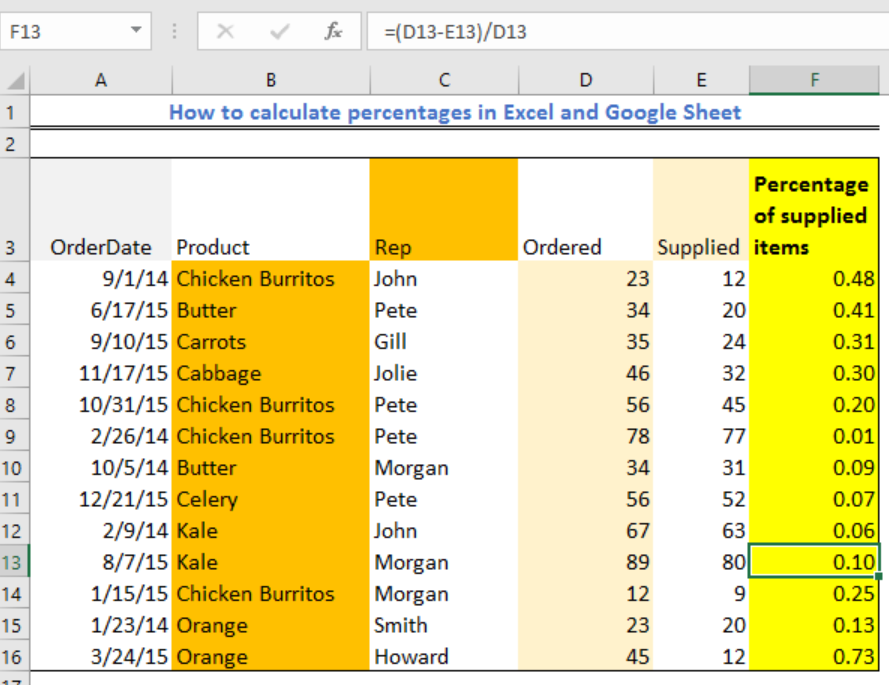Figure 3 – Calculate percentage in excel

• We will highlight the Results column
• We will navigate the Home tab to find the Number group. In this tray, we will click “Percent Style” to display our decimal fractions results as percentages.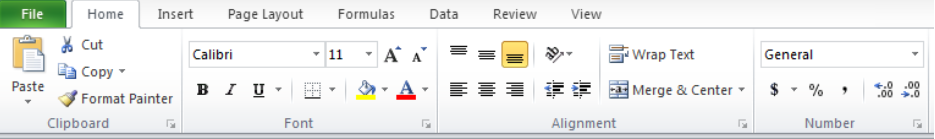Figure 4a – Click on % in Number style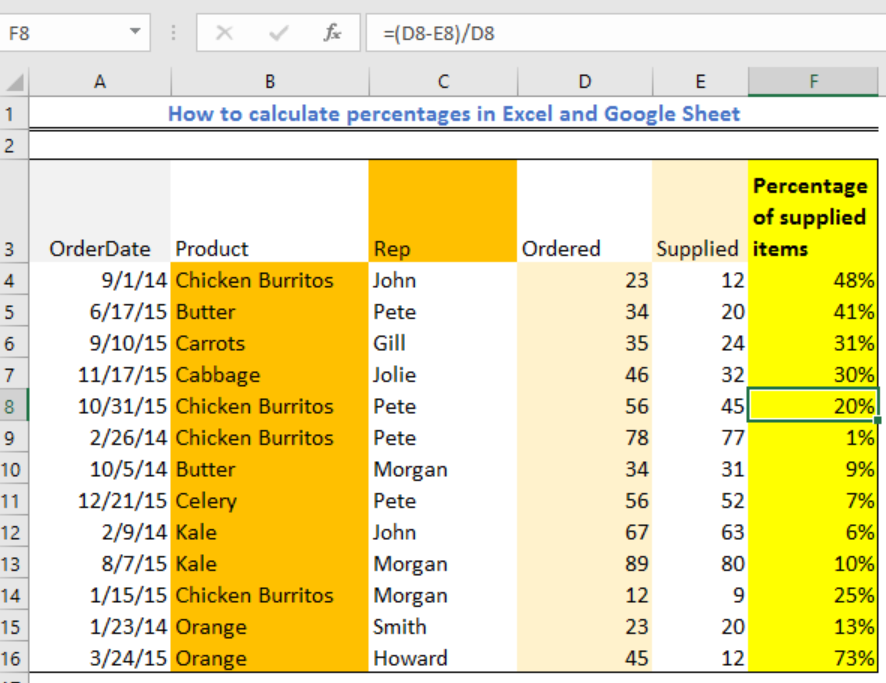Figure 4b – Excel formula for percentage increase

## Calculating the Percentage Increase (Or Decrease) Between Rows

If we wish to calculate percentage within one column of numbers like Column E, we can use these steps:

• We skip the first cell and click on Cell F5
• Next, we enter the formula below:

`=(E5-E4)/E5`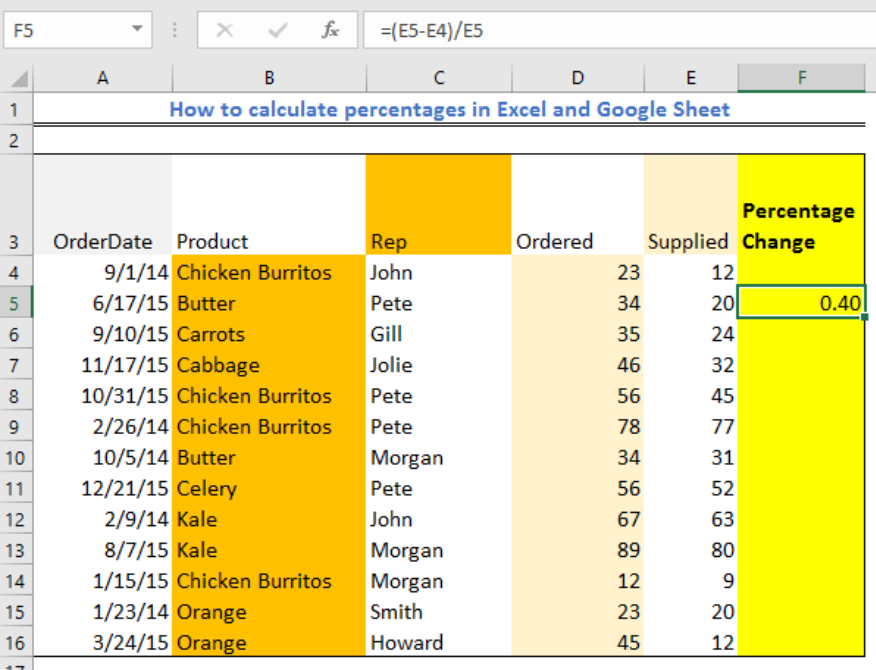Figure 5 – Percent returned formula excel

• We will use the fill handle tool to drag down the formula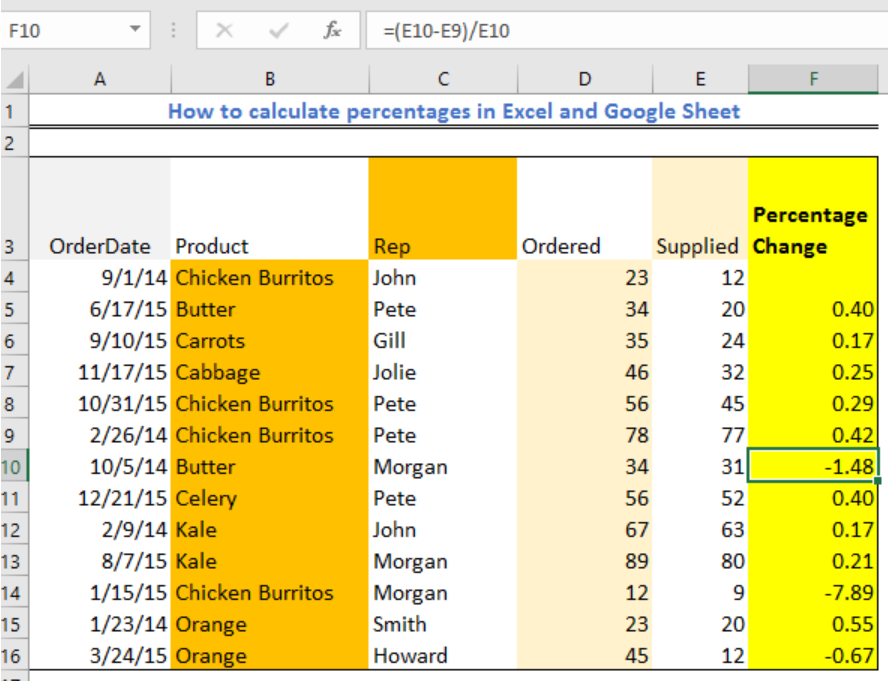Figure 6 – Percent change function excel

• We will highlight the Percentage Change column, go to the Home tab and select “Percent Style” to display results as percentages.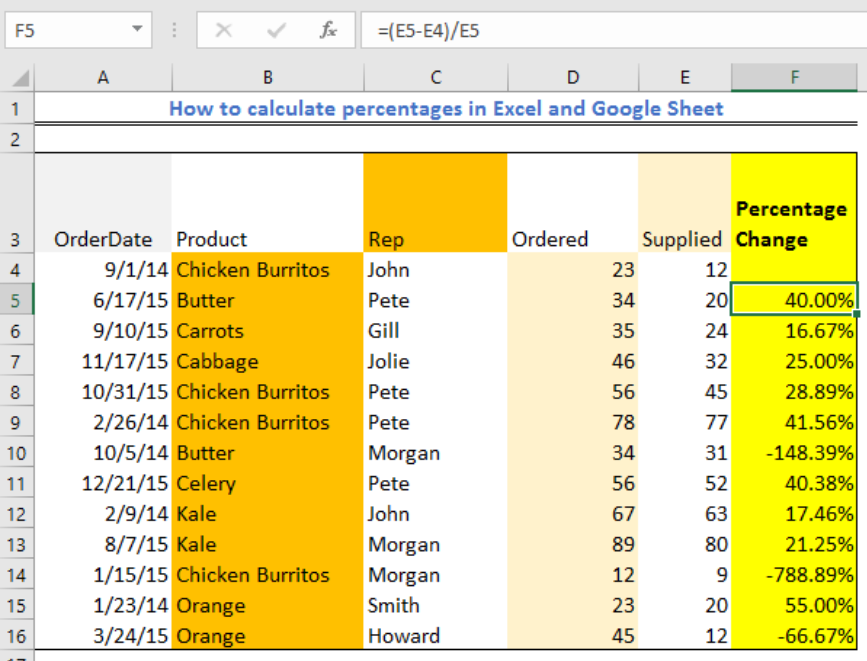Figure 7 – How to show the percentage change in excel

## Calculating Percentage Increase (Or Decrease) to a Reference Cell

We can equally use the Excel percentage change formula to calculate results to a specific cell. Here, we fix the absolute cell as e.g. \$E\$4 and then:

• We skip the first cell and Click on Cell F5, and enter the formula below

`=(D4-\$E\$4)/\$E\$4`

• We use the fill handle tool to drag down the formula.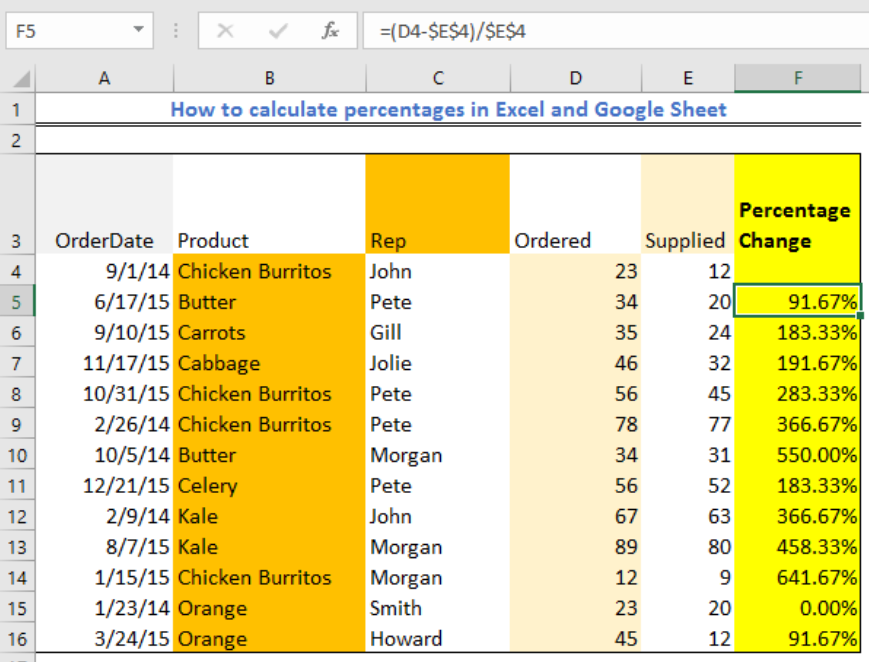Figure 8 – Excel percent change formula

• We highlight the Result column, go to the Home tab and select “Percent Style” to display results as a percentage.

## How to Calculate the Percentage of Grand total

We can see two different events when we wish to achieve Excel percent of the total. This includes:

### Total at the end of the table

When we have a Total in a single cell at the end of a Table, we simply take the Single cell as our reference cell and input this formula below. Next, we apply the percent style function

`=D4/\$D\$17`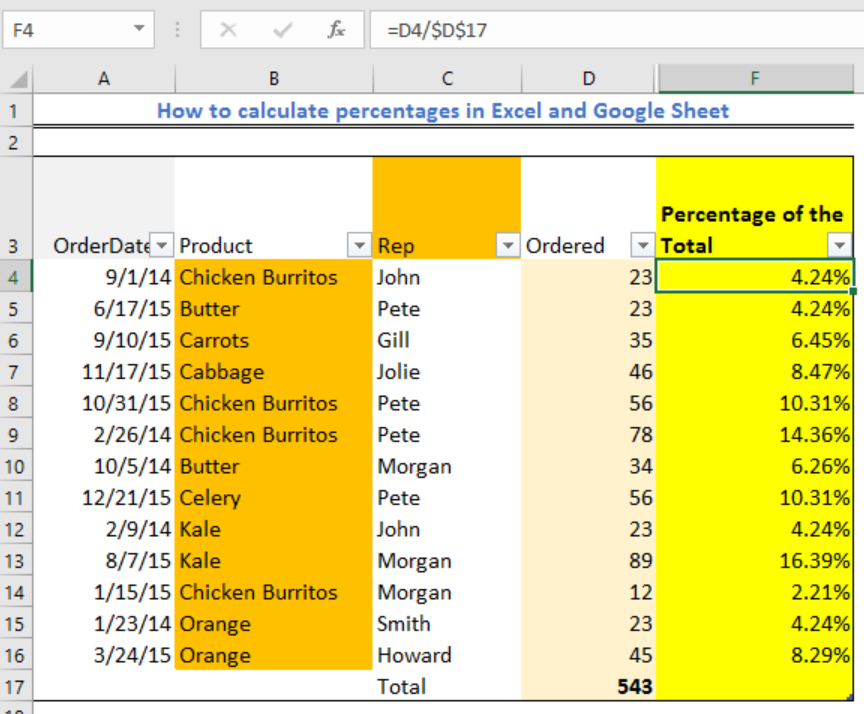Figure 9 – Excel formula for percentage increase

### Parts of Total displayed in different rows

When we have multiple rows with the same value and want to know the part of the total as a percentage, we insert the formula below in Cell F20.

`=SUMIF(B4:B16, F19, D4:D16)/(\$D\$17)`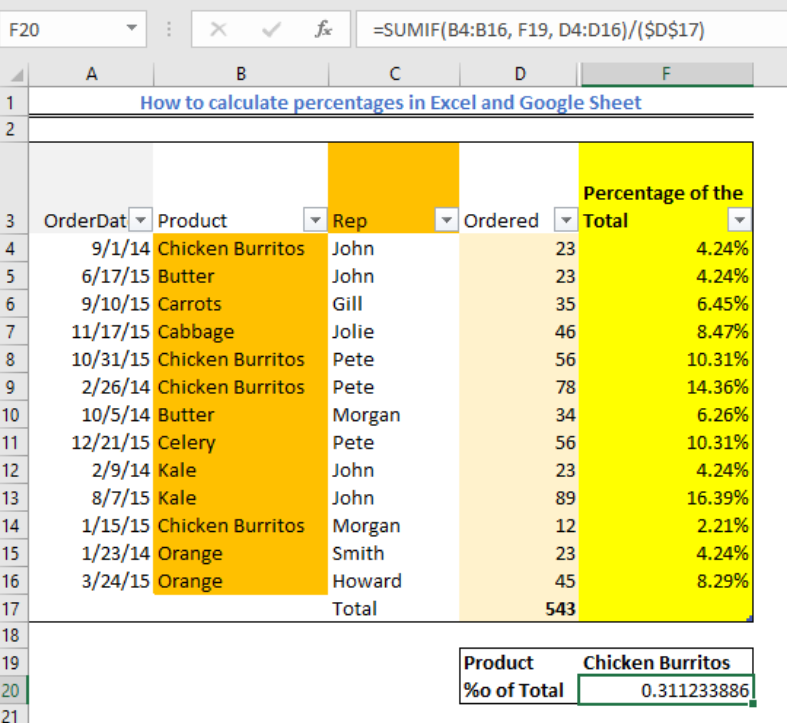Figure 10 – Parts of total derived from the Excel percentage formula

## How to Calculate the Total and Amount by a Percentage

When we know the percentage, we can quickly calculate the amount and totals easily in these steps:

### Evaluate amount by total and percentage

When we have the Total (Column A) and Percent (Column B), we can quickly learn the Amount by multiplying with the formula below:

`=A4*B4`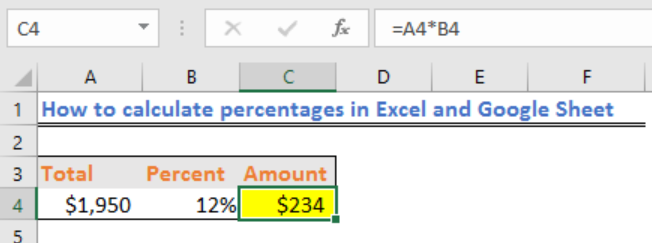Figure 11 – Excel percentage of the total result

### Evaluate total by amount and percentage

We can accuratelyequally find the total when we have the percentage and amount using this formula:

`=C4/B4` or `=C4/12%`Figure 12 – Getting total from percentage growth in excel

## Adding (or subtracting) percentage in Excel

When we wish to increase a number by a percentage in Excel across a column, without updating to a new column, we use the following steps:

• In Cell F4, we enter the excel formula to add a percentage increase or decrease

`=D4 *(1 + 20%)` (Increase by percentage) or

`=D4*(1 - 20%)` (Decrease by percentage)

• Highlight Cell F4 and press Ctrl + C to copy cell.
• Next, we highlight the column we wish to increase or reduce
• We right-click on the selection, and tap, Paste Special
• Within the Paste Special Dialog Box, we navigate to Paste and mark Values. We also mark Multiply under Operation and select OK to view results increased by 20%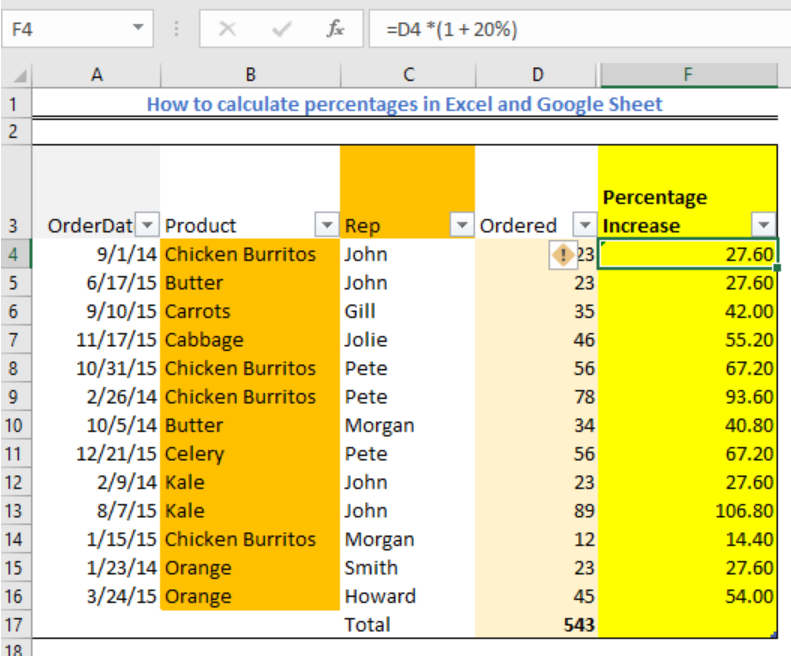Figure 13 – How to sum percentages in excel

## Multiply or Divide a column by a specified percentage

• In Cell F4, we enter one of the following formulas:

`=D4*(1 * 20%)` (Multiply by percentage) or

`= D4*(1 / 20%)` (Divide by percentage)

• Highlight Cell F4 and press Ctrl + C to copy cell.
• Next, we highlight the column we wish to increase to increase or reduce
• We right-click on the selection, and tap, Paste Special
• Within the Paste Special Dialog Box, we navigate to Paste and mark Values. We also mark Multiply under Operation and select OK to view results increased by 20%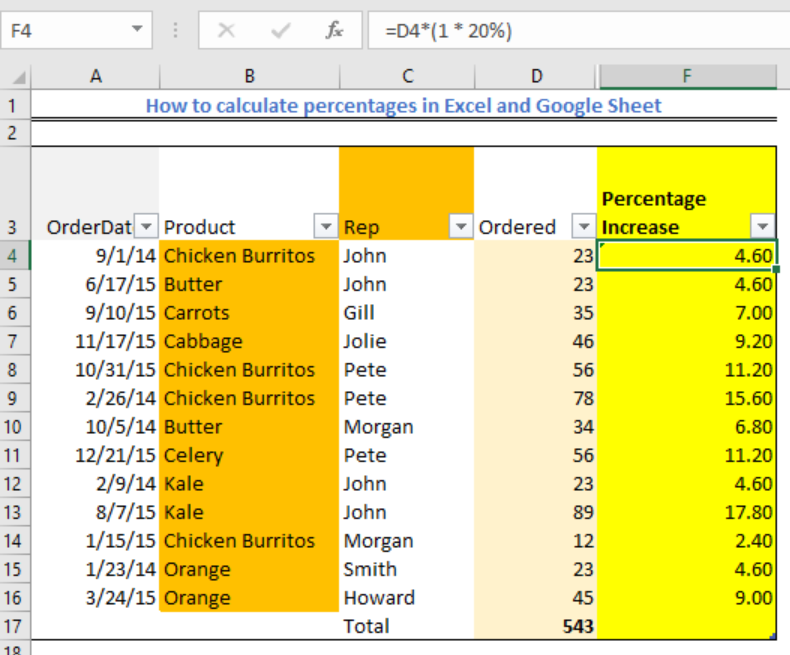Figure 14- Multiplying excel percent increase

## Using the Basic Excel Percentage formula

We use the Excel Percentage formula to calculate the percentage quickly. In the figure below, we have Column D (Ordered groceries) and Column E (Supplied Groceries). Now, we find the percentage of supplied groceries using the steps below:

• We Click on Cell F4 and enter the formula below

`=E4/D4`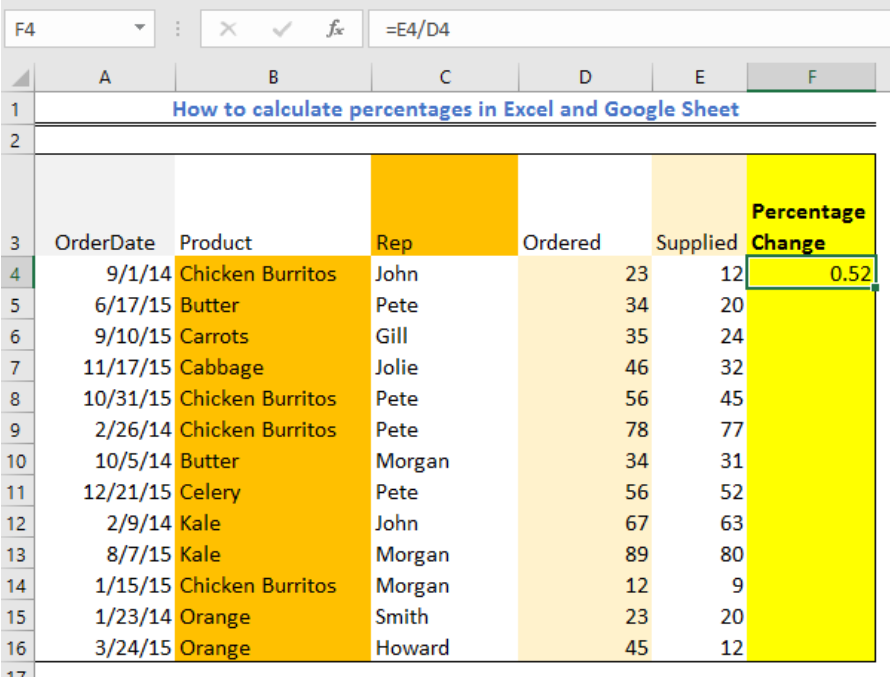Figure 15 – Basic Excel Percentage formula

• Now, we will use the fill handle tool (the small box at the lower right corner of the cell) to drag theour formula into as many rows as we need.
• Our Results will be displayed as fractions.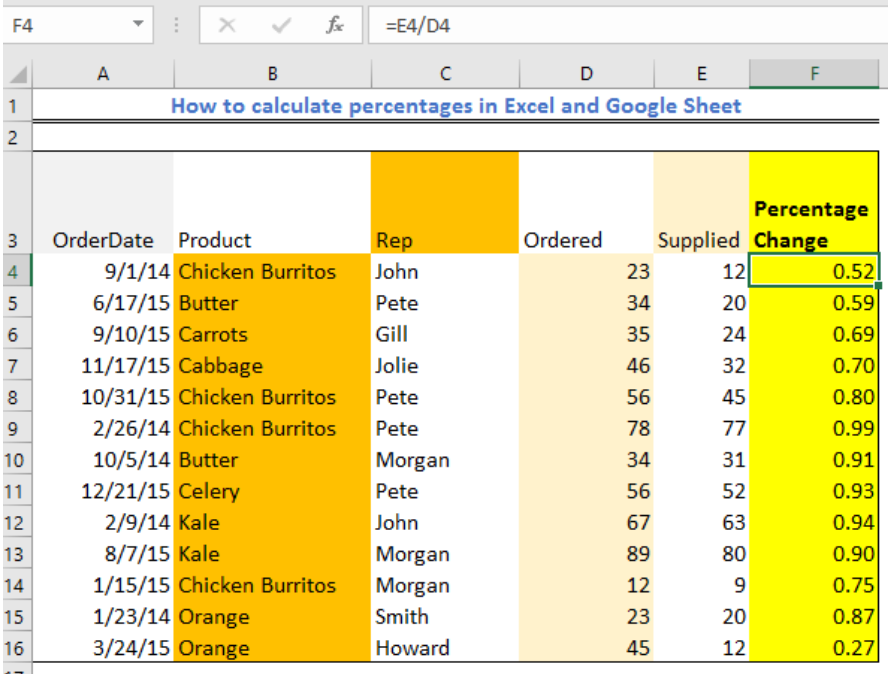Figure 16 – How to show percentage in excel

• We quickly highlight the Result column.
• Next, we navigate the Home tab to find the Number group. In this little tray, we click “Percent Style” to display our decimal fractions results as percentages.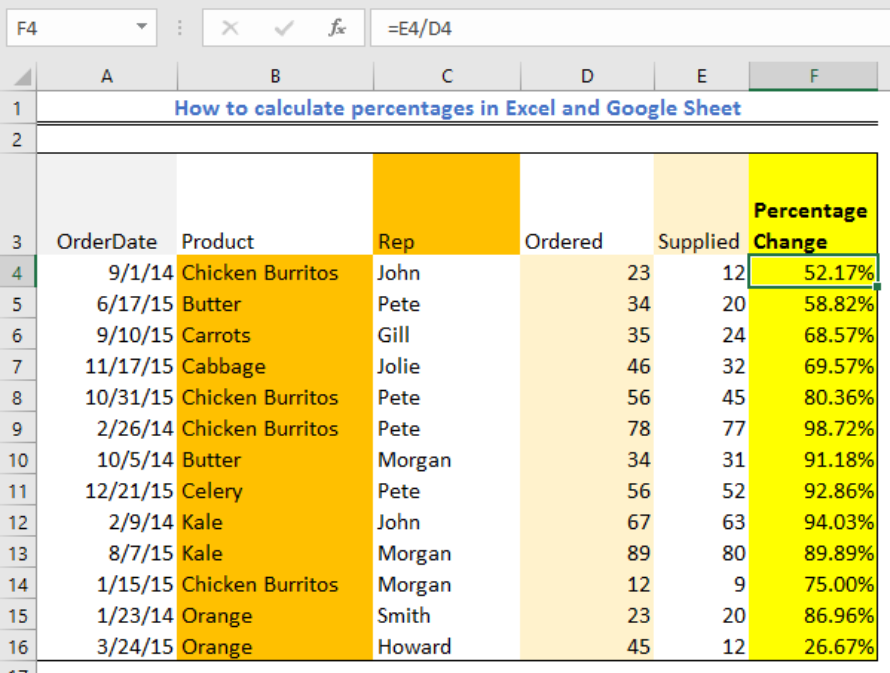Figure 17 – Calculating excel percentages.

## Note

We do not have a universal Excel percentage formula. Our approach depends on what we want to achieve. We can also see that 100 should not multiply the Basic Excel Percentage formula since Excel automatically uses the operation once we apply the percent style option from the Number ribbon.

## Instant Connection to an Excel Expert

Most of the time, the problem you will need to solve will be more complex than a simple application of a formula or function. If you want to save hours of research and frustration, try our live Excelchat service! Our Excel Experts are available 24/7 to answer any Excel question you may have. We guarantee a connection within 30 seconds and a customized solution within 20 minutes.